# How to calculation defect rate by operation of Roll throughput yield

Six Sigma – iSixSigma Forums General Forums General How to calculation defect rate by operation of Roll throughput yield

Viewing 7 posts - 1 through 7 (of 7 total)
• Author
Posts
• #53694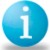thongkorn
Participant

I have a question on defect rate of roll throughput yield.
I would like to know how to calculation defect rate on roll throughput yield by operation.
Example :
Operation A : loading input 1000 pcs. found faliure part 10 pcs. , Yield = 90%
Operation B : loading input 990 pcs. found faliure part 20 pcs. , Yield = 97.9%
Operation C : loading input 970 pcs. Found faliure part 70 pcs. Yield = 92.7%
Roll thorugh put yiled = yield of operation A * yield of operation B * yield of operation C = 90.0% And defect rate = 10.0 %

My question is how to calculate the defect rate by operation.
I tried to weighted the defect by operation
Example : Total defect of operation A,B,C 100 pcs. Defect of RTY = 10 %.
Operation A : defect = (Total defect * defect of operation A)/100 = 1 %
Operation B : defect = (Total defect * defect of operation B)/100 = 2 %
Operation C : defect = (Total defect * defect of operation C)/100 = 7 %

But this calculation did not represent the actual defect of operation.
Defect by operation of operation A = 10 % Versus Defect of roll throught 1%.
The defect rate is quite different. The RTY defect is 10% which how to caluation defect that is represent the actual defect by operation.

Would you like to help suggestion and advice ?

0
#191138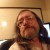Strayer
Participant

Since RTY is the product of the yields from all operations in the process it is not applicable to individual operations. Why are you trying to work backwards since you’ve already measured defect rate and yield for each operation?

0
#191157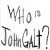MBBinWI
Participant

The first section (just after Example:) is the only thing that is needed. You identify the correct way to calculate RTY, but then show the wrong value (RTY = Yield A * Yield B * Yield C) this does not equal 90% (redo the calculation). This also does not equal a defect rate of 10% (nor does it represent the total defect rate). The RTY gives you the probability of a product being produced without any defects (there are a number of categories of defects, from actual wrong dimensions, to excessive processing, rework, inventory, etc.). For each process step, the defect rate is merely 100% – the step yield.
Hope this helps.

0
#191179Rasheed
Participant

the way u calculated is having the same results as by 100%-yeild.
its correct and also explained with correction in formula.

the total is infact 10% which is also correct and can get by adding percentages if the sample size is almost same or having very less variation in all operations. otherwise percentage addition wont work.

if the sample size is differnet then defect will be the addition of defects in all operations and sample size will be the average of all operations. and formula will be,
Def % overall = Total Def *100%/ sample size Avg

the above formula will show the defect% of overall production and for each operation below formula is correct.

operation A. Defect of A*100%/sample size of A
Operation B. Defect of B*100%/ sample size of B.

Operation C……

for 100% inspection, sample size will be the production of process.

I hope this will work

0
#203003Louise
Guest

I wonder if anyone can help. A manufacturer of water filter canisters for coffee machines supplied us with 3 faulty filters. They say they produce 30000 a year and never have any faulty goods Looking through some of posts I can see there are calculations on production runs and the percentage of defects like sigma calculation,RTY,FTY. I just need a way of stating to the company one of the calculation if there is one because I know another company that have used the manufacturer and they have had faulty goods as well. Sorry if it does not make sense.

0
#203004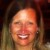Katie Barry
Keymaster

0
#203017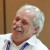Mike Carnell
Participant

Louise Sorry Katie you are right this is going back 7 years but just in case.

At the the end of the day you found 3. You didn’t order 3 defects. The supplier is not going to tell you that they have other customer that have found more. It doesn’t matter what they say.

Screen the lot and see if you find more. Send an SQE to go though FG and look. There will be more. look at sampling theory in Grant and Levenworth. You can figure out exactly what your 3 defects out of X means.

0
Viewing 7 posts - 1 through 7 (of 7 total)

You must be logged in to reply to this topic.